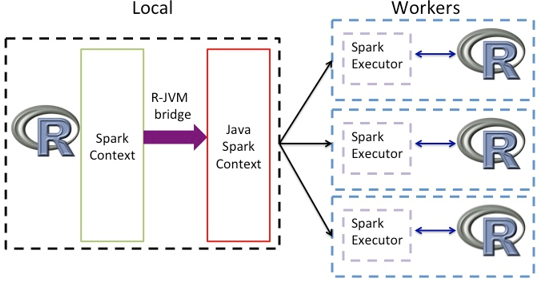# Building a real-time big data pipeline (8: Spark MLlib, Regression, R)

Published:

Updated on October 06, 2020

Apache Spark MLlib 1 2 3 is a distributed framework that provides many utilities useful for machine learning tasks, such as: Classification, Regression, Clustering, Dimentionality reduction and, Linear algebra, statistics and data handling. R is a popular statistical programming language with a number of packages that support data processing and machine learning tasks. However, R is single threaded and is often impractical to use it on large datasets. To address R’s scalability issue, the Spark community developed SparkR package4 which is based on a distributed data frame that enables structured data processing with a syntax familiar to R users.

SparkR (R on Spark) Architecture 4“SparkR is an R package that provides a light-weight frontend to use Apache Spark from R. In Spark 3.0.0, SparkR provides a distributed data frame implementation that supports operations like selection, filtering, aggregation etc. (similar to R data frames, dplyr) but on large datasets. SparkR also supports distributed machine learning using MLlib”.

The following command will start the namenode as well as the data nodes as cluster 3.

cd /Users/adinasa/bigdata/hadoop-3.2.1
$bash sbin/start-dfs.sh  Create a directory $source ~/.bash_profile
$hadoop fs -mkdir -p /user/adinasarapu  Insert data into HDFS: Use -copyFromLocal command to move one or more files from local location to HDFS. $hadoop fs -copyFromLocal *.csv /user/adinasarapu


Verify the files using ls command.

$hadoop fs -ls /user/adinasarapu -rw-r--r-- 1 adinasa supergroup 164762 2020-08-18 17:39 /user/adinasarapu/data_proteomics.csv -rw-r--r-- 1 adinasa supergroup 786 2020-08-18 17:39 /user/adinasarapu/samples_proteomics.csv  Start YARN with the script: start-yarn.sh $bash start-yarn.sh
Starting resourcemanager
Starting nodemanagers


Check the list of Java processes running in your system by using the command jps. If you are able to see the Hadoop daemons running after executing the jps command, we can safely assume that the Hadoop cluster is running.

$jps 96899 NodeManager 91702 SecondaryNameNode 96790 ResourceManager 97240 Jps 91437 NameNode 91550 DataNode  Open a web browser to see your configurations for the current session. Web UI for HDFS: http://localhost:9870 for YARN Resource Manager: http://localhost:8088 ## 2. SparkR, spark session in R environment a. Start sparkR shell from Spark installation cd to spark installation directory and run sparkR command cd bigdata/spark-3.0.0-bin-hadoop3.2/bin/ >sparkR ... ... ... Welcome to ____ __ / __/__ ___ _____/ /__ _\ \/ _ \/ _ / __/ '_/ /___/ .__/\_,_/_/ /_/\_\ version 3.0.0 /_/ SparkSession available as 'spark'. During startup - Warning message: In SparkR::sparkR.session() : Version mismatch between Spark JVM and SparkR package. JVM version was 3.0.0 , while R package version was 2.4.6  b. Install SparkR package install.packages(remotes) library(remotes) install_github(“cran/SparkR”)  c. Open RStudio and set SPARK_HOME and JAVA_HOME Check java version system("java -version") If you see an error like “R looking for the wrong java version” set Sys.setenv(JAVA_HOME=java_path) Error in checkJavaVersion() : Java version 8 is required for this package; found version: 14.0.2  Set env variables Sys.setenv(SPARK_HOME = "/Users/adinasa/bigdata/spark-3.0.0-bin-hadoop3.2") .libPaths(c(file.path(Sys.getenv("SPARK_HOME"), "R", "lib"), .libPaths())) java_path <- normalizePath("/Library/Java/JavaVirtualMachines/jdk1.8.0_261.jdk/Contents/Home") Sys.setenv(JAVA_HOME=java_path)  Load SparkR and start SparkSession which is the entry point into SparkR. library(SparkR) SparkR::sparkR.session(master = "local", sparkHome = Sys.getenv("SPARK_HOME"), appName = "SparkR", spark.executor.memory = "2g")  To list config values with keys as their names, sparkR.conf()  Read data from hadoop/csv file. In Spark 2.0 csv is natively supported so you should be able to do something like this s.df <- SparkR::read.df("hdfs://localhost:9000/user/adinasarapu/samples_proteomics.csv", source = "csv", header = "true") # s.df # SparkDataFrame[SampleID:string, Disease:string, Genetic:string, Age:string, Sex:string] # Replace Yes or No with 1 or 0 newDF1 <- withColumn(s.df, "Disease", ifelse(s.df$Disease == "Yes", 1, 0))
newDF2 <- withColumn(newDF1, "Genetic", ifelse(s.df$Genetic == "Yes", 1, 0)) # head(newDF2) # Change Column type and select required columns for model building createOrReplaceTempView(newDF2, "df_view") new_df <- SparkR::sql("SELECT DOUBLE(Disease), DOUBLE(Genetic), DOUBLE(Age) from df_view") model <- spark.glm(new_df, Disease ~ Genetic + Age, family = "gaussian",maxIter=10, regParam=0.3) # Print model summary summary(model)  Results Deviance Residuals: (Note: These are approximate quantiles with relative error <= 0.01) Min 1Q Median 3Q Max -0.68368 -0.40454 0.09583 0.39245 0.53625 Coefficients: Estimate Std. Error t value Pr(>|t|) (Intercept) 0.0408043 0.3501497 0.11653 0.908092 Genetic 0.2797034 0.1278479 2.18778 0.037519 Age 0.0084589 0.0054737 1.54538 0.133896 (Dispersion parameter for gaussian family taken to be 0.1752621) Null deviance: 6.6667 on 29 degrees of freedom Residual deviance: 4.7321 on 27 degrees of freedom AIC: 37.73 Number of Fisher Scoring iterations: 1  Further, compute predictions using training data preds <- predict(model, training)  Note: One of the major changes of Apache Spark for R version 4.0.0 (06/2020) [2.4][SPARK-31918][R] Ignore S4 generic methods under SparkR namespace in closure cleaning to support R 4.0.0+ (+18, -13)> This PR proposes to exclude the S4 generic methods under SparkR namespace in closure cleaning to support R 4.0.0+ in SparkR. Without this patch, you will hit the following exception when running R native codes with R 4.0.0 df <- createDataFrame(lapply(seq(100), function (e) list(value=e))) count(dapply(df, function(x) as.data.frame(x[x$value < 50,]), schema(df)))

org.apache.spark.SparkException: R unexpectedly exited.
R worker produced errors: Error in lapply(part, FUN) : attempt to bind a variable to R_UnboundValue


Other important Spark Dataframe Operations: filter, select, summarize, groupBy, arrange(=sort)

head(filter(s.df, s.df$Age > 50)) head(select(s.df, s.df$SampleID, s.df$Genetic)) head(select(filter(s.df, s.df$Age > 55), s.df$SampleID, s.df$Disease))
head(summarize(groupBy(s.df, s.df$Disease), mean=mean(s.df$Age), count=n(s.df$Genetic))) head(arrange(s.df, asc(s.df$Age)))


Combine Spark DataFrame operations using library magrittr

library(magrittr)
f_df <- filter(s.df, s.df$Age > 50) %>% groupBy(s.df$Disease) %>% summarize(mean=mean(s.df$Age))  SQL like queries: First register Spark Dataframe as sql table createOrReplaceTempView(s.df, "df_view") new_df <- SparkR::sql("SELECT * FROM df_view WHERE Age < 51")  Convert Spark Dataframe to R DataFrame: as.data.frame or collect r.df <- SparkR::as.data.frame(new_df) # r.df <- SparkR::collect(new_df) r.df  Finally, shutting down the HDFS You can stop all the daemons using the command stop-all.sh. You can also start or stop each daemon separately. $bash stop-all.sh
Stopping namenodes on [localhost]
Stopping datanodes
Stopping secondary namenodes [Ashoks-MacBook-Pro.2.local]
Stopping nodemanagers
Stopping resourcemanager
`